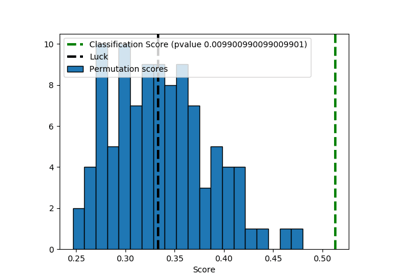/scikit-learn

# sklearn.model_selection.permutation_test_score

`sklearn.model_selection.permutation_test_score(estimator, X, y, groups=None, cv=’warn’, n_permutations=100, n_jobs=None, random_state=0, verbose=0, scoring=None)` [source]

Evaluate the significance of a cross-validated score with permutations

Read more in the User Guide.

Parameters: `estimator : estimator object implementing ‘fit’` The object to use to fit the data. `X : array-like of shape at least 2D` The data to fit. `y : array-like` The target variable to try to predict in the case of supervised learning. `groups : array-like, with shape (n_samples,), optional` Labels to constrain permutation within groups, i.e. `y` values are permuted among samples with the same group identifier. When not specified, `y` values are permuted among all samples. When a grouped cross-validator is used, the group labels are also passed on to the `split` method of the cross-validator. The cross-validator uses them for grouping the samples while splitting the dataset into train/test set. `scoring : string, callable or None, optional, default: None` A single string (see The scoring parameter: defining model evaluation rules) or a callable (see Defining your scoring strategy from metric functions) to evaluate the predictions on the test set. If None the estimator’s default scorer, if available, is used. `cv : int, cross-validation generator or an iterable, optional` Determines the cross-validation splitting strategy. Possible inputs for cv are: None, to use the default 3-fold cross validation, integer, to specify the number of folds in a `(Stratified)KFold`, An object to be used as a cross-validation generator. An iterable yielding train, test splits. For integer/None inputs, if the estimator is a classifier and `y` is either binary or multiclass, `StratifiedKFold` is used. In all other cases, `KFold` is used. Refer User Guide for the various cross-validation strategies that can be used here. Changed in version 0.20: `cv` default value if None will change from 3-fold to 5-fold in v0.22. `n_permutations : integer, optional` Number of times to permute `y`. `n_jobs : int or None, optional (default=None)` The number of CPUs to use to do the computation. `None` means 1 unless in a `joblib.parallel_backend` context. `-1` means using all processors. See Glossary for more details. `random_state : int, RandomState instance or None, optional (default=0)` If int, random_state is the seed used by the random number generator; If RandomState instance, random_state is the random number generator; If None, the random number generator is the RandomState instance used by `np.random`. `verbose : integer, optional` The verbosity level. `score : float` The true score without permuting targets. `permutation_scores : array, shape (n_permutations,)` The scores obtained for each permutations. `pvalue : float` The p-value, which approximates the probability that the score would be obtained by chance. This is calculated as: `(C + 1) / (n_permutations + 1)` Where C is the number of permutations whose score >= the true score. The best possible p-value is 1/(n_permutations + 1), the worst is 1.0.

#### Notes

This function implements Test 1 in:

Ojala and Garriga. Permutation Tests for Studying Classifier Performance. The Journal of Machine Learning Research (2010) vol. 11

## Examples using `sklearn.model_selection.permutation_test_score`Test with permutations the significance of a classification score